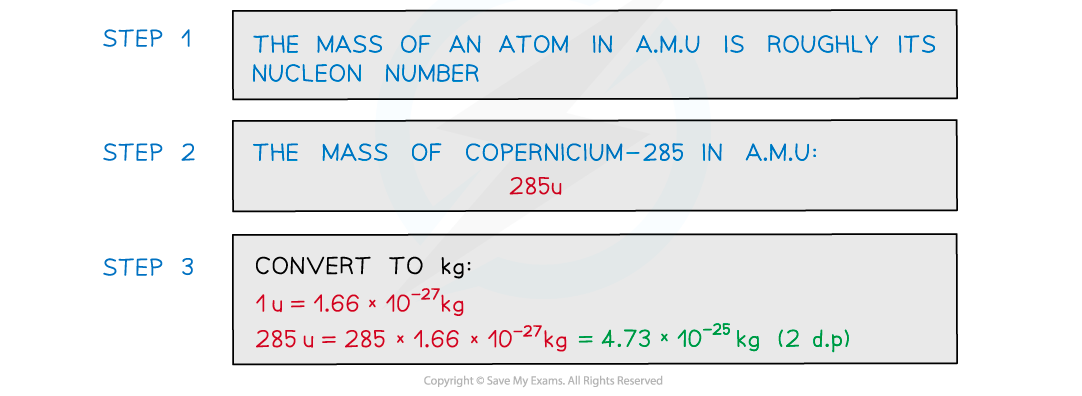# IB DP Physics: HL复习笔记7.2.1 Atomic Mass Unit

### Atomic Mass Unit

• The unified atomic mass unit u is roughly equal to the mass of one proton or neutron:
• 1u = 1.66 × 10−27 kg
• It is sometimes abbreviated to a.m.u
• This value will be given on your data booklet in the exam
• The a.m.u is commonly used in nuclear physics to express the mass of subatomic particles.
• It is defined as:#### Worked Example

This question concerns nuclear mass:

a) Estimate the mass of the nucleus of element copernicium-285 in kg.

b) Using the values for proton and neutron mass given in the data booklet, determine the total mass of all components for a nucleus of element copernicium-285 in kg.

Part (a)Part (b)

Step 1: Identify the components of copernicium-285

• Since the element is copernicium which is the 112th element, this atom must have 112 protons
• Therefore, it will have:

285 – 112 = 173 neutrons in its nucleus

Step 2: Determine the amount of atomic mass units for these components

• The proton's mass can be found by multiplying the number of them by their mass in amu which is given in the data booklet:

112 × 1.007276 = 112.814912 u

• The neutron's mass similarly can be found using their relevant amu mass:

173 × 1.008665 = 174.499045 u

Step 3: Combine the amu mass of the protons and neutrons

• This combination is a mass of:

112.814912 + 174.499045 = 287.313957 u

Step 4: Convert the amount of mass from amu to kg

• The conversion is done using the value:
• u = 1.66 × 10−27 kg

287.313957 × 1.66 × 10−27 = 4.7694116862 × 10-25 kg

Step 5: State the final answer to 5 decimal places

• The final answer for the total mass of all components of nucleus of element copernicium-285 is approximately:

4.76941 × 10-25 kg

#### Exam Tip

In most of the IB DP Physics course, significant figures would be expected to be rounded to 2 or 3 digits as the situation or problem deems appropriate. In topic 7, Atomic, Nuclear, and Particle physics, often you will need to continue to answer to 6 significant figures. This is because the level of precision can and will change an answer to a nuclear physics question for different levels of rounding.